# JavaScript Quirks: Numbers Edition

As a developer working with JavaScript, perhaps you’ve run into “huh?” moments from working with what some characterize as quirks of the language. On the face of it, each of the statements below presents an unexpected outcome. But if you dig deeper into how the datatypes and APIs are implemented, you’ll find there is always a reason behind these quirks.

`0.1 + 0.2 !== 0.3; // output: true`
`parseInt('2/888') !== parseInt(2/888); // output: true`
`[100,1, 2, ].sort(); // output: [1, 100, 2]`

So, how many of these quirks belong to the JavaScript language, and how many are representative of Computing Science in general? In this post we’ll go deep into how JavaScript works with numbers so that you can avoid the most common mistakes you might make when working with Numbers in JS.

### What is a JavaScript number?

The Number data type is one of the 7 JavaScript data types. The other 6 being:

1. String
2. Boolean
3. Undefined
4. Null
5. Symbol
6. Object

The object data type is the only non-primitive data type. It includes dates, objects, and arrays, etc.
The number data type is just as diverse. Numbers in JavaScript include integers, floats, binaries, hexadecimal… just to name a few. Examples of JavaScript numbers include:

1. `123245`
2. `1.234`
3. `Infinity, -Infinity, 0`
4. `0x31333131 // hexadecimal`
5. `0232 // octal`
6. `0b1111 // binary`
7. `1.2e-1 // scientific notation`
8. `NaN`

NaN is also a number! That sounds counterintuitive, yet it is true. To verify `NaN` is a number, enter `typeof NaN` in a terminal and press enter. The output is `"number"`. `NaN` is a popular concept in Computing Science and is prevalent in many programming languages. It simply means a unrepresentable number, yet it is represented like any other JavaScript number.

### What is not a JavaScript number?

Not everything that looks like a number is a number. Any String is not a number even when it looks like one. For example `‘1’` is not a number because `typeof '1'` is `"string"`.
A BigInt is another Number lookalike; it looks like an integer with ending n. For example `0n`, `-4325325n`, and `12345568574324546574534n` are all BigInts. As the name suggests, BigInt only works for integers. BigInt accurately represents integers, since not all integers can be represented using a JavaScript Number. BigInt also looks, works, and is represented differently from numbers. This means that during BigInt to Number conversions, you risk potential data loss.

## How are JavaScript numbers represented?

Glad you asked! JavaScript numbers are represented using double precision floating point standard, specifically the IEEE 754 floating point standard. That’s a lot of terminology! What do double precision and floating point mean?
Double precision means 64 bits to store each number. All else being equal, having more bits to store a number means being able to store more numbers accurately, so 64 bit storage means a bigger range than 32 bit storage. Some typed languages have the option to define a number as a float (32 bits) or double (64 bits). In JavaScript, all numbers, from floats to integers to special numbers, are represented in 64 bits.
What about floating point representation? Some programming languages such as Apex, C, and C++have the concept of int, in addition to float and double. Stored differently from floating point, examples of ints would include `1000` or `2` . However, in JavaScript, all numbers are stored using the 3 part floating point regardless of whether it has a decimal point or not.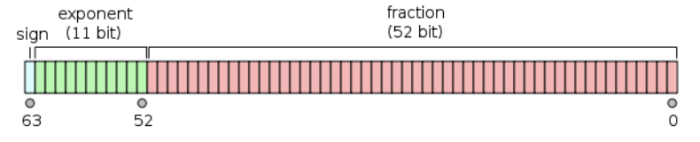Let’s go over the three parts one by one

1. Signed bit: 0 for positive, 1 for negative
2. Exponent: how large or small the number is. A multiplier for the fraction
3. Significand/base/fraction: used for more precise numbers. These bits represent increasing negative powers of 2

### Floating point representations

Let’s see a few floating point representations for an overview of how it works.

0’s representation is the most minimalist and the smallest binary possible. For negative numbers the signed bit will be 1.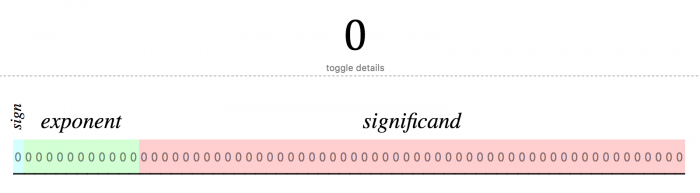For 1, notice the exponent bits go up to 011 1111 1111. In base 10 that binary is 1023. 1023 is important because if the exponent is smaller than that the number represented is between absolute one and zero. If the exponent is larger than that, the number represented is larger than 1.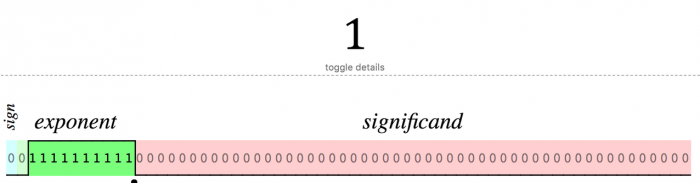For -1, notice the representation is identical to that of positive 1, except for the flipped signed bit.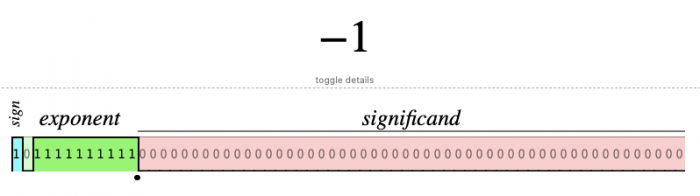For simplicity let’s go back to positive numbers. Take 1’s representation and increment the exponent by one to get 2. Notice the larger the exponent, the larger the number.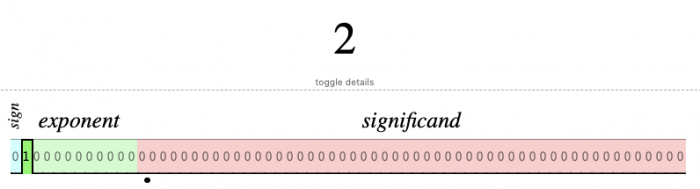What about floats? 1.5 is a sum of 1 + 0.5, so its representation reflects that. Because significands are increasingly negative powers of 2, the first bit in the significand represents 1/2, and is flipped to 1.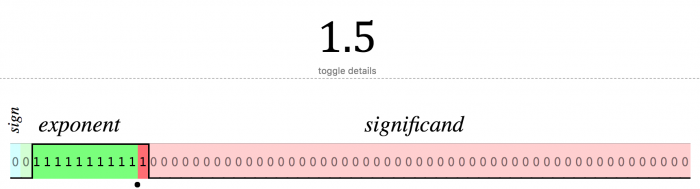1.75 is a sum of 1 + 0.5 + 0.25, so its representation reflects that. Note that the second bit in the significand represents 1/4, and is flipped to 1.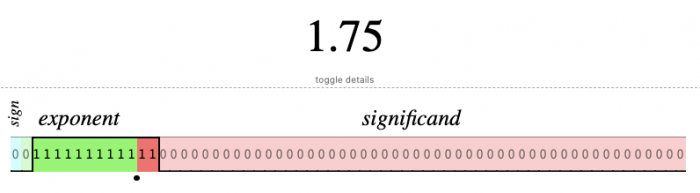How does one represent `Infinity` with a finite number of bits? Since `Infinity` is a super large number, it makes sense to flip all the 1’s in the exponent to 1.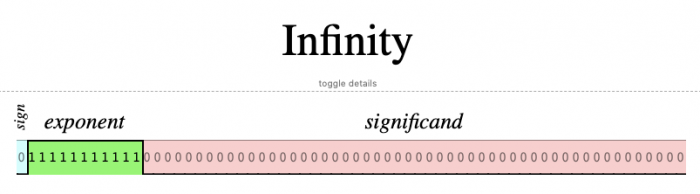What is beyond Infinity? If you enter `Infinity + 1` you get `NaN`! Here is the unrepresentable number represented just like any other number. Notice its representation is `Infinity + 1/2`.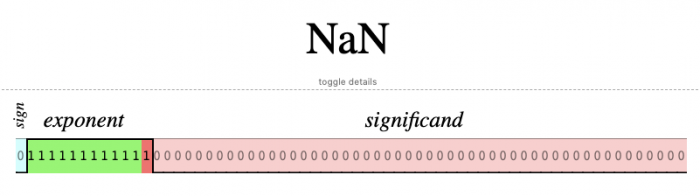How does one represent the largest safe integer? See below. Notice that all of the bits in the significand are flipped to 1, and the floating point is at the end of the 64 bit register.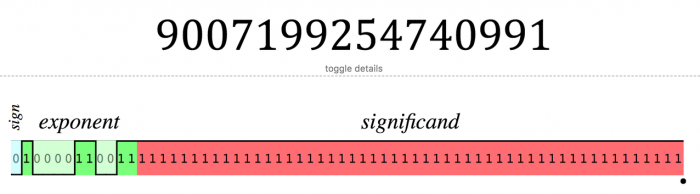What happens when we increment the `NUMBER.MAX_SAFE_INTEGER` by 1? the floating point is floating off of the 64 bits, a clear indicator that the number is unreliably represented. `9007199254740992` is unreliably represented because both itself and `9007199254740993` map to the same representation. The further the floating point is off, the more bits are missing, thus the more likely the number is mis-represented. There are no errors: JavaScript silently fails to represent very large and small numbers.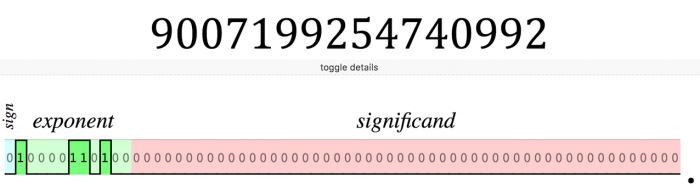### When does the number representation fail?

The representation will silently fail for very small or very large numbers, because those numbers need more than 64 bits to be accurately represented. Their 64 bit representation is unreliable and potentially inaccurate.
There is a safe range to represent integers: Integers from `-Math.pow(2, 53) + 1` to `Math.pow(2, 53) - 1` inclusive have 1:1 mapping between the number and its representation. Within this range, the integer is accurately represented. When outside of this range, consider using BigInt to accurately store integers.
To test out whether `yourNumber` is within the safe range, use `Number.isSafeInteger(yourNumber)` . The method outputs yes for integers smaller or equal to `Number.MAX_SAFE_INTEGER`, and no for larger integers and floats.
Unfortunately, there is no equivalent method to test the safeness of floats. Also you cannot use BigInt to represent floats, since BigInt only represents integers.

## Quirky JavaScript number handling

The floating point representation is a Computing Science concern, and the ensuing quirks are prevalent across programming languages such as Apex, C++, Java, and Python. In addition to floating point quirks, JavaScript also exhibits quirky behavior through its built-in methods. Let’s go over two popular gotchas.

### Array.prototype.sort(optionalFunction)

The out of the box `Array.prototype.sort(optionalFunction)` is simple: it sorts the elements in increasing order and modifies the underlying array. It sorts a string array, but does it sort a numbers array?
For example what is the output of `const arr = [100, 1, 2]; arr.sort();` If it was sorted in ascending order we can expect `[1, 2, 100];` However the result is different!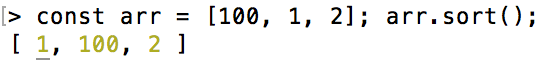The result is unsorted and differs from the original! What is going on? According to the official EMA spec on sort, since we omitted the comparator function, each element in the array is converted to a string then compared using the Unicode order. That’s how we got the `[1, 100, 2]` result. Lesson learned! Always pass in a comparator function when dealing with number arrays. More details in the docs.

parseInt is deceptively simple. Put in a string or number and get an integer out, right? A basic example of it working is `parseInt('2'); // outputs 2`

Let’s start with the optional second parameter. What if you omit the radix (aka the base)? In other words, would these outputs be identical? `parseInt('0x32')` vs `parseInt('0x32', 10)`
The only difference is that the second code snippet has 10 as the radix. If you think the default radix is base `10`, then the results should be the same. But they differ! What is going on?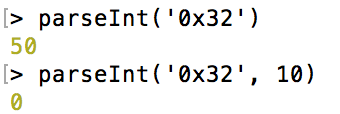In the first code snippet, parseInt looks at the string and deduces that the underlying number is hexadecimal, since the string starts with `0x`. Since `32` in hexadecimal is `3 * 16 + 2`, parseInt returns `50`. For the second example, parseInt has the same string input, but `x` is not in base 10, so everything from `x` onwards is discarded. Hence the result is `0`.
Since the results differ, supply the radix to avoid surprises.

String vs number
Moving onto another parseInt quirk: does parseInt treat the string input and number input equally? One might assume that since parseInt accepts both string and number, that it should treat them equally. So `parseInt('2/5556789', 10)` should have the same output as `parseInt(2/5556789, 10)`.
Again the results differ. Let’s deconstruct what happened here.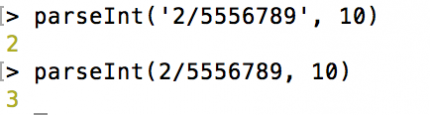In the first code snippet, parseInt looks at the string `'2/5556789'`. Because the `‘/’` character is not in base 10, all characters from there onwards are discarded, and `2` is returned. In the second code snippet, the first parameter is a number. The scientific notation for this number is `3.5992009054149796e-7`, since large and small numbers have a tendency to be converted to scientific notation. parseInt correctly parses out 3 from that.
Since the results differ from string to number, use parseInt with strings and avoid passing in numbers to it. To get integers from numbers, use `Math.round(number)` for consistent and predictable results. In our example, `Math.round(2/5556789)` correctly returns `0`.

## Summary

There are plenty of quirks in JavaScript and plenty of quirks with numbers. This article scratched the surface of what can be quirky about JavaScript numbers, namely the parseInt and Array.prototype.sort. Use a comparator function for the sort and always supply a radix with parseInt.

The inaccuracy of floating point representation is separate from JavaScript. The double precision representation is limited whenever the number requires more than 64 bits for accurate representation. Large and small numbers are prone to inaccuracies. Only numbers whose binary format is finite can be represented accurately with finite bits. Suffice to say, floating point approximates numbers.

Now you know everything about numbers! Take the quiz and see how well you do!
Warning: the quiz might not be as easy as you think. Feel free to study the resources below before taking the test 🙂

### Resources

Documentation
Products
Developer Centers
Learn
Community